# Projectile Motion-how far can one throw a javelin?

• Author:
• Updated date:Javelin throwing, an example of projectile motion in athletics. (source: corbisimage.com)

Have you ever thrown a javelin? If so, why you throw it at the angle of around 450? - instead of say 30 or 60. Of course, because experience teaches us that the range will be maximal at that angle. But is there some fundamental, physical reason explaining that fact? If not deal with javelin, for sure you threw a stone as a child, might competing with your friends who would throw it more away.

Let discussing one more example of projectile motion. A rescue plane spots a lost mountaineers and have to throw them emergency rations. In which moment the plane should throw a package: when it is just above a mountaineers, a several hundred meters before that point or once it passed the mountaineers?

In this article we are going to study physics underling such a motions, that are called projectile motions. More precisely, projectile motion is a motion caused by gravity. Usually, when studying physics of these motions there are two essential assumptions. Firstly, we suppose that motions are close to the Earth's surface, which means that the acceleration of gravity force is constant. Second assumption is that there is no any air resistance. Although these assumptions are close to reality, especially the first one, the difference between theoretically calculated trajectory (taking into account these two simplification) and real trajectory could be significant.

## Background: Uniformly Accelerated Motion, Principle of Independence of Motion

Before starting with the subject it worth to repeat two background physics. Two very important lessons in this sense are uniformly accelerated motions and the principles of independence of motion. Briefly, if a body performs more than one motions than these motions (or components of the motion) are independent. This means they don't influence each other. For example, if two coins are falling from the edge of table they, one directly and another horizontally will grounded in the same time. Namely, the coin performing complex motion will approach to the ground as it hasn't horizontal component of velocity.

Regarding uniform accelerated motion, let us mention that there are two essential expressions, one defining acceleration (a=v/t) and another one for the distance traveled (s=v*t+ 1/2at2). All other equations within uniformly accelerated motion are some combination of the two. This is very brief reminder indeed, since a comprehensive explanation would be out of the scope of this article.

## Vertical Projectile

Firstly we will study two special types of projectile and finally a generalization will be given. The first interesting case is when a projectile is thrown vertically with some initial velocity.

The physical situations is illustrated on the first figure. The projectile is thrown vertically with the initial velocity v0from the height h0. Let now answer a few interesting questions.

What is the speed of the projectile in the point B? - So, the projectile starts with v0 and than gradually lose its velocity until the point B where the velocity is 0.

What is the acceleration of the projectile in the point B? - This question is little bit harder than the previous one; the acceleration in B is equal to g, the acceleration of gravity.

What is the speed of the projectile in the point C? - the same as in the point A i.e. v=v0.

In what time the projectile will reach the point B? - It depend only on initial velocity: g=v0/t which means t=v0/g.

What is the maximal height, h-h0? - Here we simply apply the equation v=sqrt(2as) for the uniform accelerated motion where v is velocity, a is acceleration and s is the distance traveled. Thus, h-h0=v0 2/2g .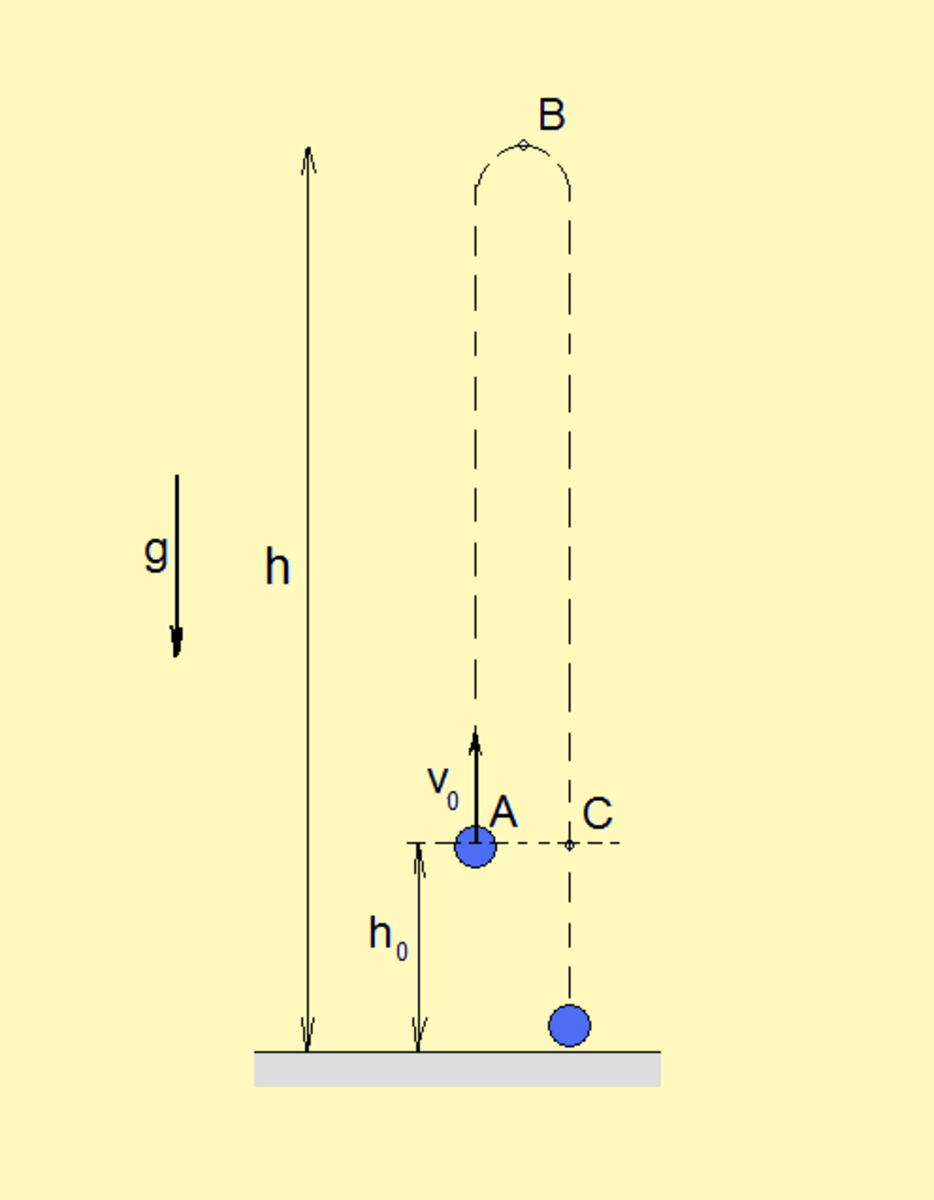Vertical projectile. This motion is composed by uniform motion and free fall. (source: flysky)

Now we approach to the more complex questions. As we already comment, the speed will gradually decline from v0in A to 0 in B. We want to know what is the velocity and any particular moment t. In case there were no gravity in the moment t the velocity of the body will be v0 (according to he First Newton's low). In case there would be only gravity, without the initial velocity, the velocity in the moment t will be g*t. Applying the principle of motion independence this means that in the moment t it holds

v=v0 -gt. (1)

Similarly, we ask what is the h of the projectile in the moment t. Analogically, in the moment t uniform motion (caused by the initial velocity) contributes with v0*t whilegravity contribute with gt2/2. Finally, it gives the relation

Scroll to Continue

h = h0 + v0t - gt2/2. (2)

## Horizontal Projectile

In case of a projectile that is horizontally thrown with a certain initial velocity, there are two components of motions. Horizontally, the body move all the time with constant speed. In the same time, the body performs free fall. As we know, the two motions are independent on each other. One of the most interesting question is what is the distance D (see figure) that the body will passes before it landed. This distance is usually called the horizontal range of the projectile. It is worth mentioning that the trajectory here will be a parabola. The vertex of parabola is in the starting point of the body.

Intuitively, we see that D depend on the initial velocity v0, on the height h0, and of course on gravitational acceleration g. As a first step towards an answer, let discussing the next questions. Does the time needed for projectile to landed depend on v0?

No! That time don't depend on the initial velocity but only on the value of h0. Moreover, this time is the same as the time of free falling body to lend from the height h0. Thus, having known h0 it holds h0= ½ * g t2, which leads to t=sqrt(2h0/g).

Now with ease one can write a final equation. Due to the uniform motion in horizontal direction, it holds v0=D/t. Then D=v0*t. Finally, putting the previous expression for t, it holds D=v0*sqrt(2h0/g).

Returning to the question form beginning of the article, now we know precisely where or when the package have to be thrown in order to fall precisely within the lost mountaineers. We have to know the height on the plane as well as its velocity. Then we calculate the distance D. The package should be thrown in the distance D before the goal. Surely, in a real case we have to know a position of mountaineers.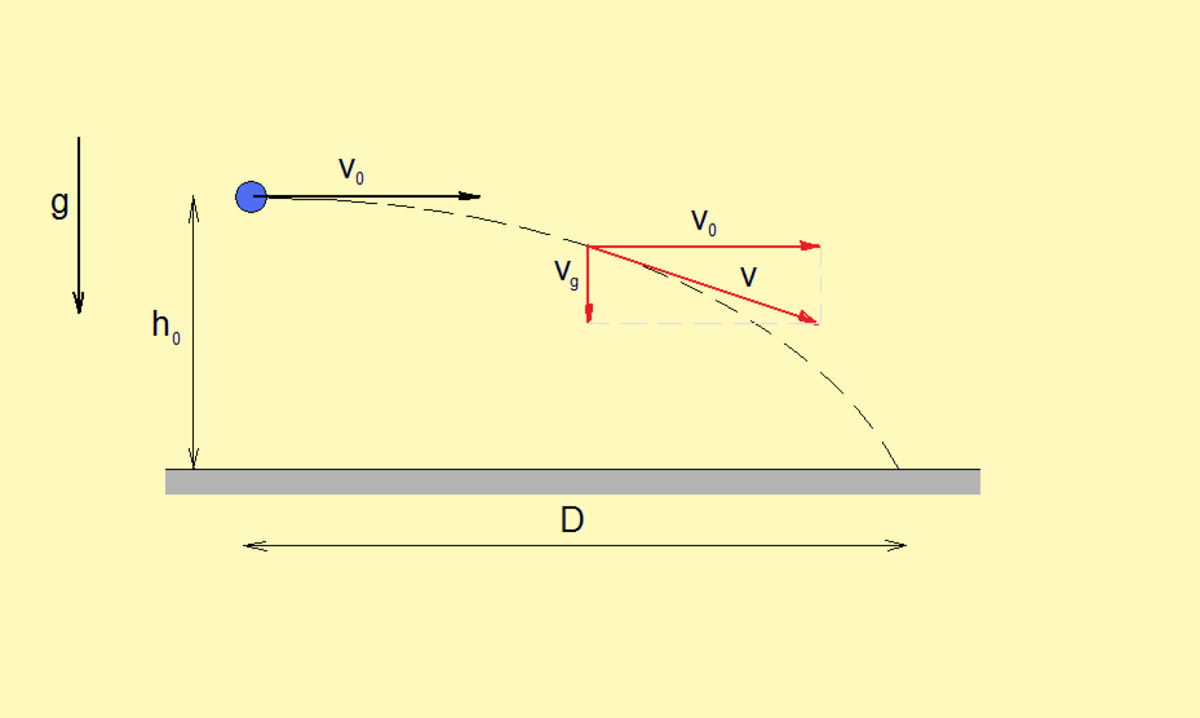Horizontal projectile, a nice illustration of the principle of independence of motions. (source: flysky)

## Projectile Motion

Until now we study two special cases of projectile motion, when a body is thrown vertically and horizontally. In the first case we can say that the body is thrown by an angle of 900, whereas in the case of horizontal motion the angle is 00. Surely, a projectile can be thrown by any other angle between the two values (see the figure).

Generally, there are three components of the projectile motion: uniform motion horizontally, uniform motion up and uniform accelerated motion down. Having this in mind, we can quite easily derive the equations, although this general case is the most complex among the our three cases. Thus, the components of initial velocity are v0x=v0 cos(α) and v0y=v0 sin(α).

Substituting these equations into equations (1) and (2) gives the velocity components and position coordinates of the projectile at the time t.

vx= v0x =v0 cos(α) (3)

vy=v0y -gt = v0 sin(α)-gt (4)

x= v0x t = v0 cos(α) t (5)

y = v0yt - gt2/2 = v0 sin(α)t - gt2/2 (6)

The trajectory here will be a parabola, just as in the case of horizontal projectile. Apparently, its vertex is in the highest position of the projectile. This point is a special one of the motion, having only horizontal component of the velocity. Furthermore, coordinates of the projectile are symmetric with respect to this point (which is clear once we know that parabola is symmetric). The same symmetry holds for the time of motion; half of the total motion time the projectile will spend for reaching the peak and the other half from the peak to the ground.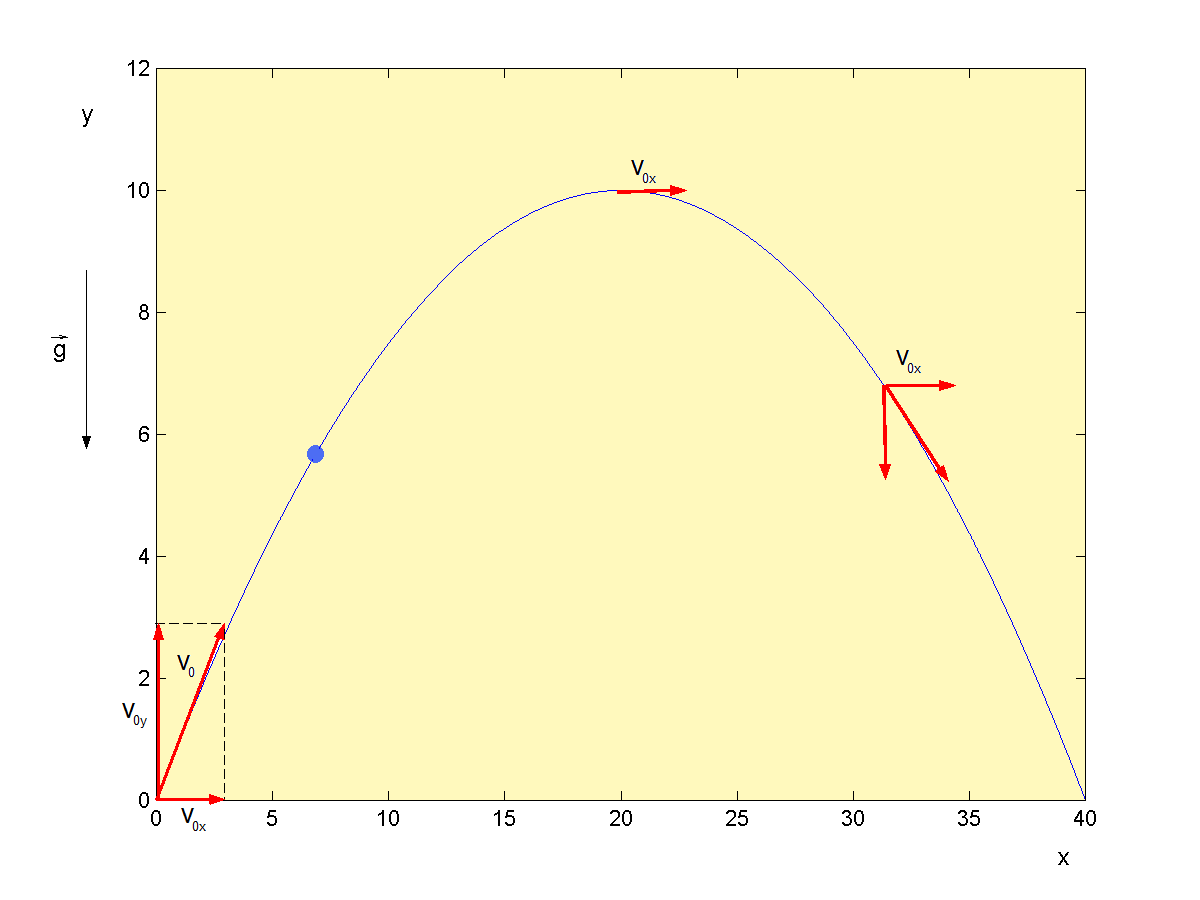Projectile motion. Two basic assumptions within projectile motions are: gravitational acceleration is constant and we neglect the air resistance. (source: flysky)

## Horizontal Range and Maximum Height of a Projectile

Equation (4) can be used to determine the time T the body takes to reach the peak. Namely, in that point worth vy = 0. Furthermore, it holds

T= v0 sin(α) / g (7)

Let now derive the expression for the maximal height H the body reach. Substituting the expression (7) in equation (6) we have

H = v0 sin(α)t - gt2/2 = v0 sin(α) * v0 sin(α) / g - (g/2) * (v0 sin(α) / g)2= v02 sin2(α) - v02 sin2(α) /2

H= v02 sin2(α) /2 (8)

Finally, it remain to derive the equation for horizontal range D of the projectile. Once having equations 3-6 this would be an easy task. So, x= v0x t = v0 cos(α) t . If the body needs the time T to reach the peak, it needs 2T to reach the ground. Thus,

D = v0 cos(α) 2T = v0 cos(α) 2 (v0 sin(α) / g ) = 2 v02sin(α) cos(α) /g

It is known that sin(2α) = 2 sin(α) cos(α). Finally we usually write the expression for D in a form

D=v02sin(2α) /g (9)

## Video 2: Projectile Motion Summary

 t/s y/m 1 9.1 2 8.2 0.2 2.6 1.4 9.9 0.6 6.6 2.2 6.9 2.6 2.9 2.8 0.3

## Case Studies

Goolkeeper's kick

A boy threw a ball by the velocity 8 m/s and the angle of 450 in respect to the horizontal. When the ball reach the height of 8 meters first time and when for the second time?

In order to have an insight into the matter, let draw a graphs representing the motion. In the same time, the diagram y - t gives the solution directly. According to the equation (6) we have a function, y(t) = v0 sin(α)t - gt2/2 . Obviously, the ball reach the height in 0.8 th second for the first time and slightly after 2nd second for the second time. The expression (4) enable us to draw the vy-t diagram of the motion. On this diagram one can see a vertical velocity of the ball at any moment.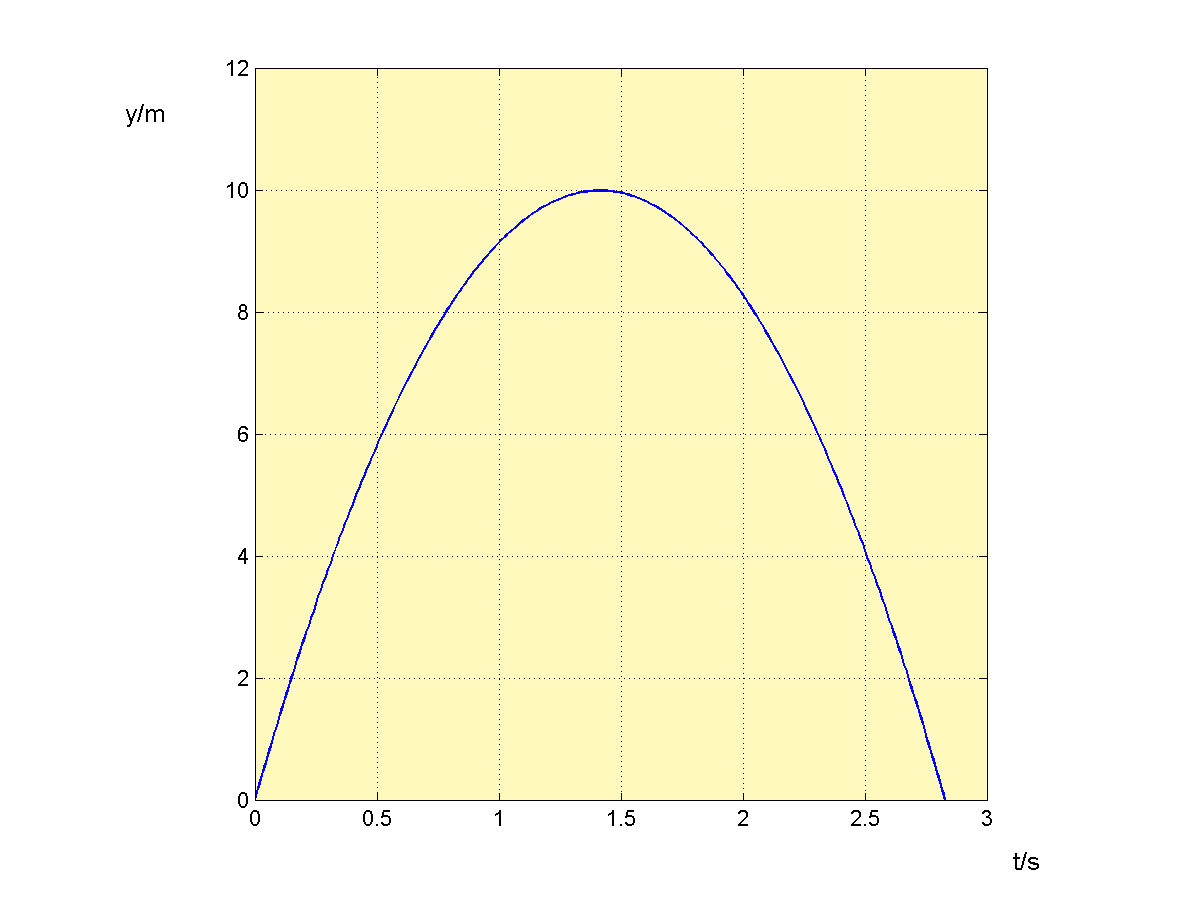y - t diagram, showing the graph of function y(t) = v0 sin(α)t - gt2/2. (source: flysky)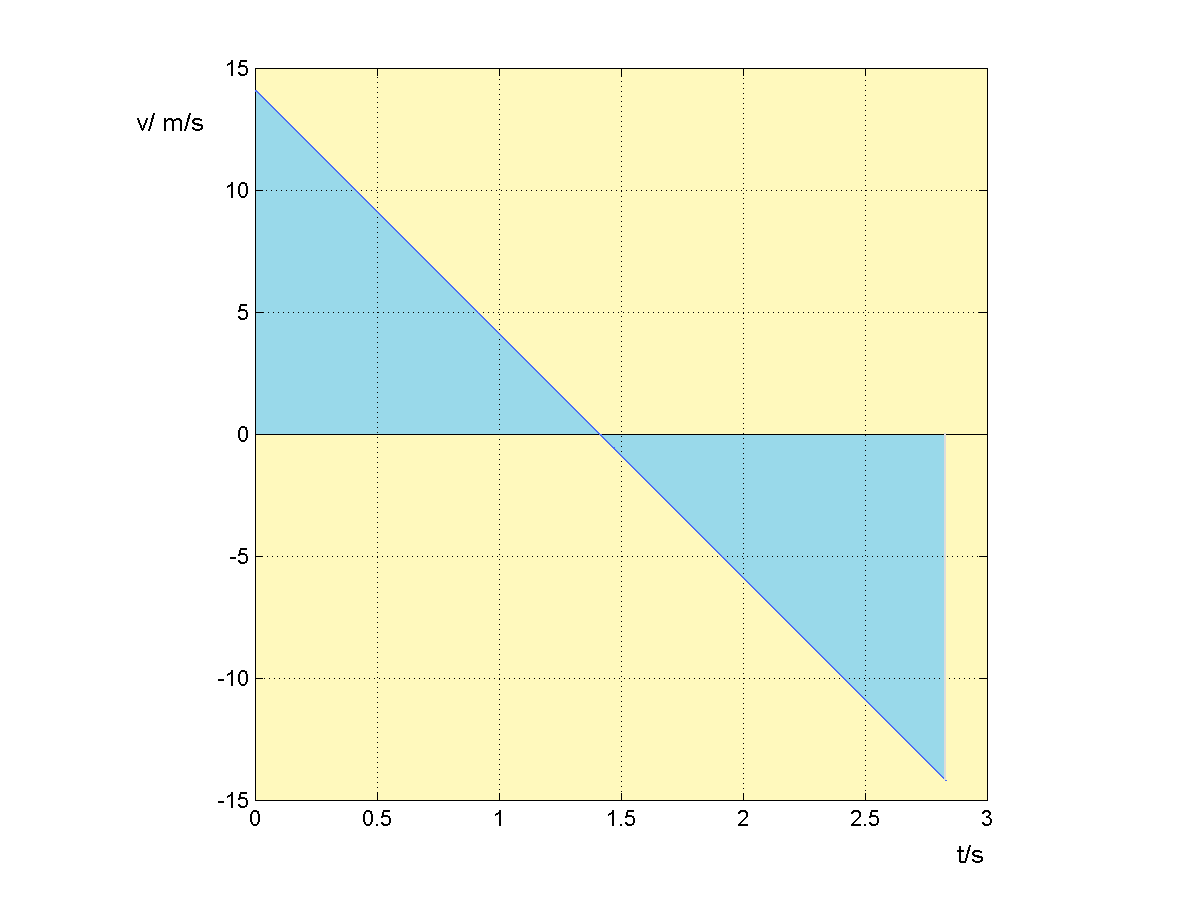v - t diagram, showing graph of the function v(t)= v0 sin(α)-gt. (source: flysky)

For each question, choose the best answer. The answer key is below.

1. Two coins, on the edge of the table are thrown at the same time, but one directly and another horizontally to the floor.
• The first coin will fall first.
• The second coin will fall first.
• Coins will fall in the same time.
2. In the example of rescue plane and mountaineers when the package should be thrown?
• Exactly above the mountaineers.
• When they were in sight.
• Once the plane passes the mountaineers.
3. If the initial height within horizontal projectile increases 4 times. How this would impact the horizontal range?
• Horizontal range will remain the same.
• Horizontal range will increase double.
• Horizontal range will increase 4 times.
4. Why the maximal horiznotal range is by 45 0?
• Because sin(90 0)=1
• Because sin(45 0)=1/sqrt(2).
• Because cos(90 0) = 0.
5. What would be the trajectory of the ball by goalkeeper shoots, if we ignore air resistance?
• Ellipse
• A curve similar to parable
• Parable

1. Coins will fall in the same time.
2. When they were in sight.
3. Horizontal range will increase double.
4. Because sin(90 0)=1
5. Parable

An aerostat at 400 meters

From an aerostat, which is located at an altitude of 400 meters drop a ball. Calculate the time the ball needs to fall to the ground if:

a) aerostat rises vertically by the velocity 10 m/s,

b) aerostat descend vertically by the velocity 10 m/s,

c) aerostat suspended in the air,

d) aerostat moves horizontally by the velocity 10 m/s.

Firstly, note that results for c) and d) will be the same. The ball in that cases performs pure free fall, so it holds H (400 m) = ½ g t2. After a little calculation result of 8.9 second follows.

One possible way to solve the fist task, a), is to calculate the time the ball need to reach the peak. Then we simply calculate the time for free fall from that altitude. Concretely, the ball need 1 second to reach the altitude of 5 meters. The fall from 405 meters lasts approximately 9 seconds. For the task b) we have h = v0t +gt2/2 which results with 8 seconds.

BIJAY KUMAR from INDIA on December 09, 2019:

Good knowledge of projectile motion.

flysky (author) from Zagreb, Croatia on July 06, 2015:

Many thanks Larry! Yes, I also found this subject impressive!

Larry Rankin from Oklahoma on July 06, 2015:

Very interesting analysis. Love looking at the physics of various sports.

flysky (author) from Zagreb, Croatia on November 09, 2013:

...yes, the nature is much more complex than we can describe it by formulas. Here, the equations perfectly work if there is no atmosphere (and g is constant), which is not the case on the Earth i.e. it is not our regular experience. I put javelin in the article both as motivation for the subject and an (imperfect) example of projectile motion. So, for practical purposes these formulas need certain “corrections”. But, still it would be hard to describe all your techniques by formulas...

Mr-Klued-Up on November 09, 2013:

I am a 70+ meter javelin thrower found your hub too full of empty formulas give examples on how these physics equations are used in actual tested values.